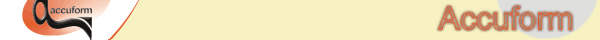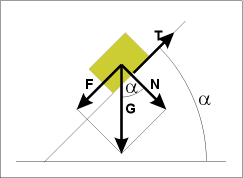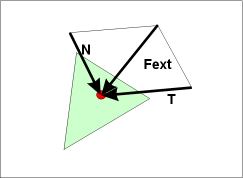T-SIM V4.5 Reference

Q1: What values should I use for heat transfer and friction ?
Q2: How is friction coefficient defined ? How is friction implemented in T-SIM ?
Q3: I want to use material different from those presented in the T-SIM material database. What can I do ?
Q4: My sheet goes through the tool. What is wrong ?
Q5: Solution ends with "Too big deformation" message. What can I do ?
Q6: I have calculated cooling, but all the sheet remains red and I can see no temperature changes.
Q7: My sheet does not deform, even when using a high pressure value.
____________________________________________________________

Q1: What values should I use for heat transfer and friction ?
A1: See Heat transfer and friction.

Q2: How is friction coefficient defined ? How is friction implemented in T-SIM ?
A2: Friction coefficient as implemented in T-SIM is given by a ratio of tangent and normal force:Suppose we have a solid body laying on a plain with slope alpha: It is obvious that F / N = tg alpha. When F = T (T is friction force), the body starts to slide. Then we can calculate the friction coefficient as f = tg (alpha) = T / N.

Following table shows friction coefficient values for different angles:

 Angle alpha (°) Friction coefficient 0 0 25 0.47 45 1 89 57.3 90 infinity

Implementation in T-SIM:The red point is a sheet node in a contact with a tool element painted in green. Fext force is acting on the sheet node due to loads on the element (pressure, gravity etc.). Tangent (T) and normal (N) components of the Fext are calculated, the calculation is based on the angle between Fext and the tool element plane. Having T and N components, we can decide whether the node will slip or not: If N . f >= T the sheet node will not slide, if N . f < T the sheet node will slide.

Q3: I want to use material different from those presented in the T-SIM material database. What can I do ?
A3: Regarding material tests and material model fit, see Material measurement and Material data fitting. Contact Accuform or local Accuform representative if you need to perform material testing and / or material data fitting.

Q4: My sheet goes through the tool. What is wrong ?
A4: When having problems with the contact detection, make sure the tools are used in the right way (see Process control settings).

Q5: Solution ends with "Too big deformation" message. What can I do ?
A5: Make sure the sheet temperature corresponds to the material reference temperature (if there is too big difference between sheet and material reference temperature, instabilities can appear).

Q6: I have calculated cooling, but all the sheet remains red and I can see no temperature changes.
A6: During the cooling calculation, the temperature scale has fixed limits. To get a scale with the real temperature minimum and maximum after the calculation, click on Limits button on the Scale bar and select Automatic in the Limits group.

Q7: My sheet does not deform, even when using a high pressure value.
A7: Check the sheet thickness - if it is too big, the sheet is too stiff to deform. Check also the sheet temperature changes during simulation. When the sheet cools down, it is getting stiffer.